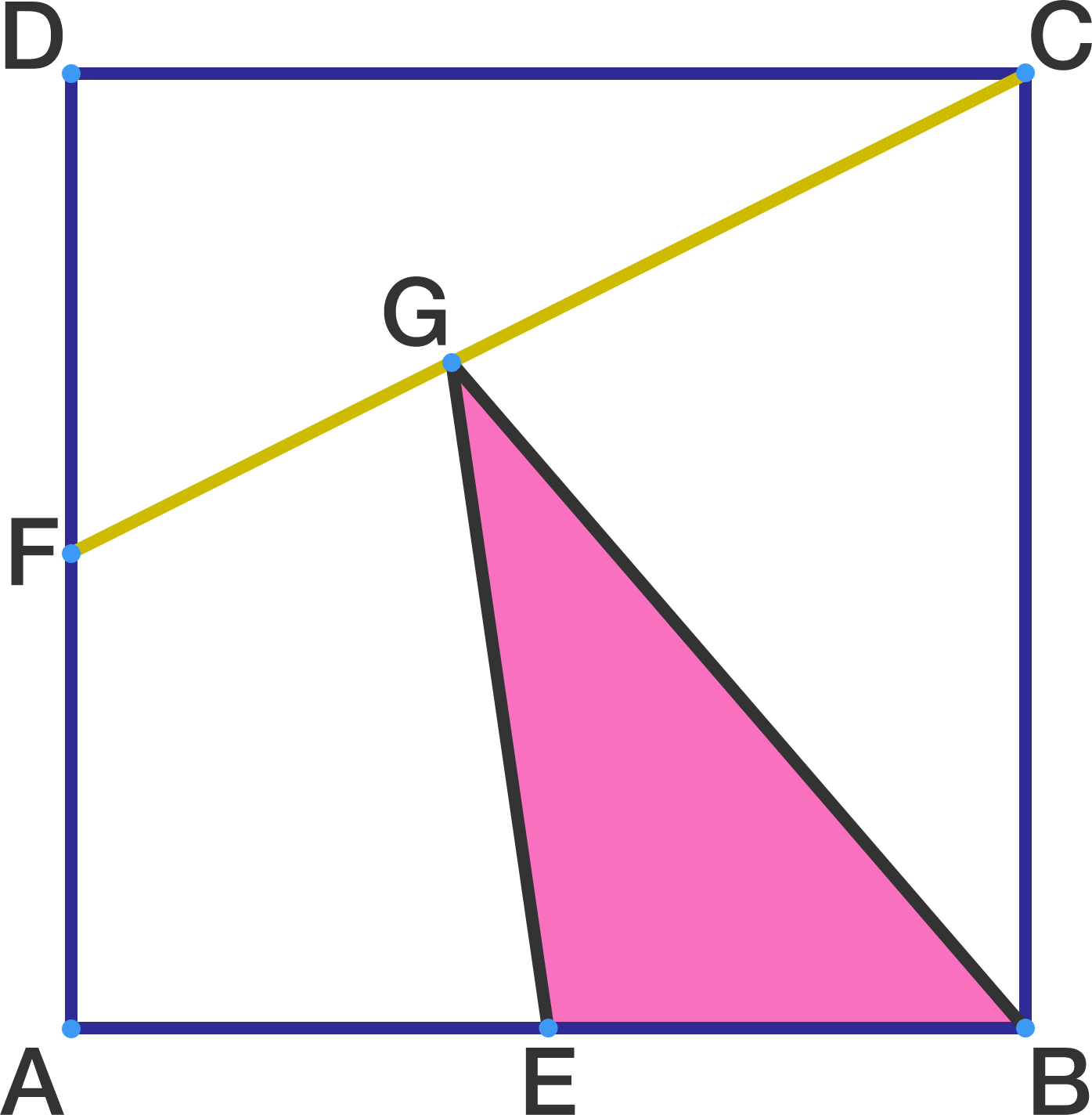# A geometry problem by Isaac Arce Aguilar

Geometry Level 2In a square $ABCD$, $E$ and $F$ are the midpoints of sides $AB$ and $AD$, respectively. A point $G$ is taken on segment $CF$ in such a way that $2CG=3FG$.

If the side of the square is $2$, then what is the area of $\triangle{BEG}$?

×